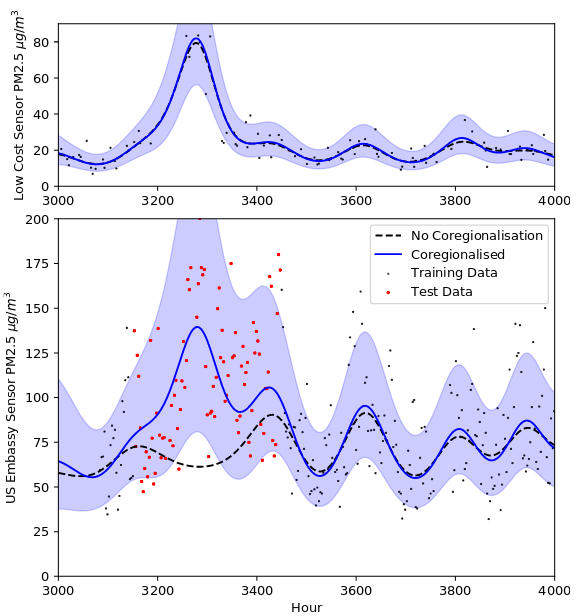Air pollution coregionalised between the US embassy and Makerere campus

Next week I’ll be presenting at Manchester’s Advances in Data Science conference, on the air pollution project. I’ve written an extended abstract on the topic.

We use a custom-crafted kernel for coregionalising between multiple sensors, to allow us to make probabilistic predictions at the level of the high-quality reference sensor, across the whole city, using the low-quality noisy sensors. We estimate the coregionalision parameters using training data we’ve collect – which ideally should include close or colocated measurements from pairings of sensors.

In future we hope to:

1. Include the uncertainty in the coregionalisation (e.g. by integrating over the distribution of the coregionalisation parameters, e.g. using CCD.
2. Allow this coregionalisation to vary over time. This will require non-stationarity, and is probably best achieved using a more flexible, non-analytic solution. E.g. writing the model in STAN
3. .

4. Updating the model in real time. I think another advantage of using a STAN or similar framework would be the gradual inclusion of new MC steps incorporting new data, as we throw out old data, this allows the gradual change of coregionalisation to be incorporated.

## Update

We can’t just use the standard coregionalisation kernel, as we’re not just kronecker-product multiplying a coregionalisation matrix with a repeating covariance matrix. Instead we want to element-wise multiply a matrix that expresses the coregionalisation with another matrix that expresses the covariance due to space and time proximity (see above figure).

Here is the GPy kernel code to do this;

```import GPy
import numpy as np
from GPy.kern import Kern
from GPy.core.parameterization import Param
#from paramz.transformations import Logexp
#import math
class FlatCoreg(Kern):
"""
"""

def __init__(self, input_dim, active_dims=0, rank=1, output_dim=None, name='flatcoreg'):
super(FlatCoreg, self).__init__(input_dim, active_dims, name)

assert isinstance(active_dims,int), "Can only use one dimension"

W = 0.5*np.random.randn(rank,output_dim)/np.sqrt(rank)
self.W = Param('W', W)
self.link_parameters(self.W) #this just takes a list of parameters we need to optimise.

if X2 is None:
X2 = X.copy()

dK_dW = np.zeros([self.W.shape,X.shape,X2.shape])
for i,x in enumerate(X):
for j,x2 in enumerate(X2):
wi = int(x)
wj = int(x2)
dK_dW[wi,i,j] = self.W[0,wj]
dK_dW[wj,i,j] += self.W[0,wi]

def k_xx(X,X2,W,l_time=2.0,l_dist=0.1):
#k_time = np.exp(-(X-X2)**2/(2*l_time))
#k_dist = np.exp(-(X-X2)**2/(2*l_dist))
k_coreg = coregmat[int(X),int(X2)]
return k_coreg #k_time * k_dist * k_coreg

def K(self, X, X2=None):
coregmat = np.array(self.W.T @ self.W)
if X2 is None:
X2 = X
K_xx = np.zeros([X.shape,X2.shape])
for i,x in enumerate(X):
for j,x2 in enumerate(X2):
K_xx[i,j] = coregmat[int(x),int(x2)]
return K_xx

def Kdiag(self, X):
return np.diag(self.K(X))

k = (GPy.kern.RBF(1,active_dims=,name='time')*GPy.kern.RBF(1,active_dims=,name='space'))*FlatCoreg(1,output_dim=3,active_dims=2,rank=1)
#k = FlatCoreg(1,output_dim=3,active_dims=2,rank=1)
#k.coregion.kappa.fix(0)
```

This allows us to make predictions over the whole space in the region of the high quality sensor, with automatic calibration via the W vector.